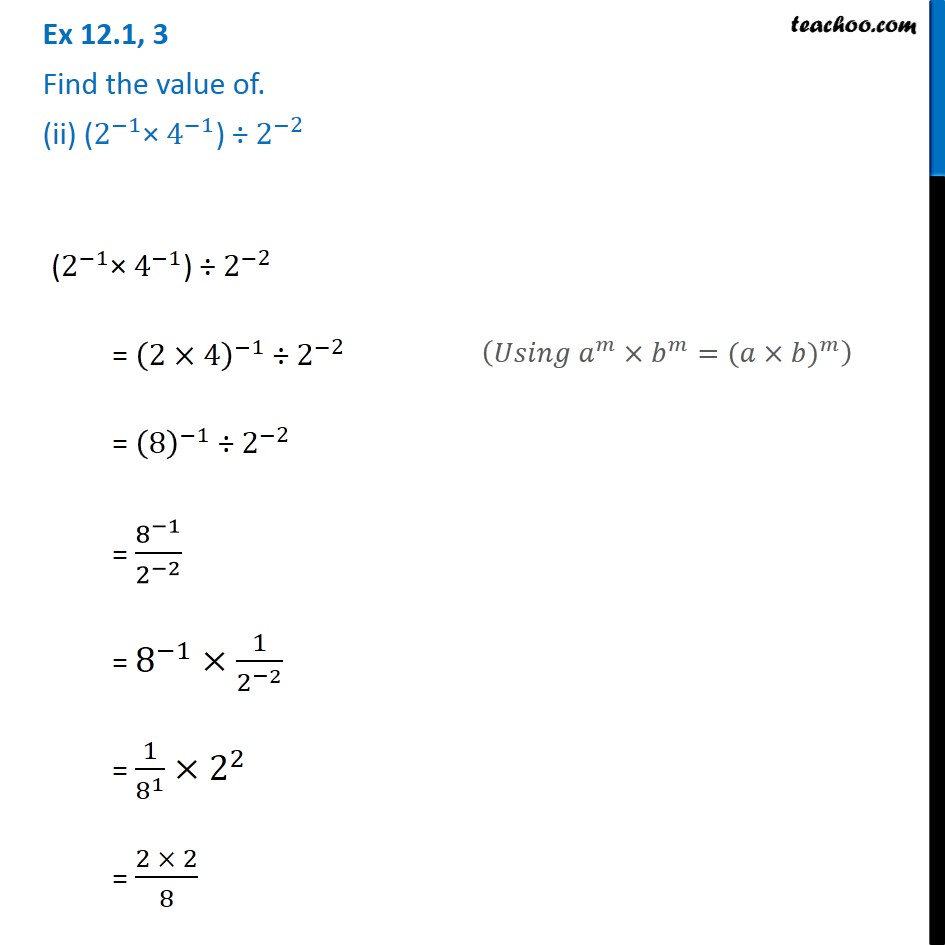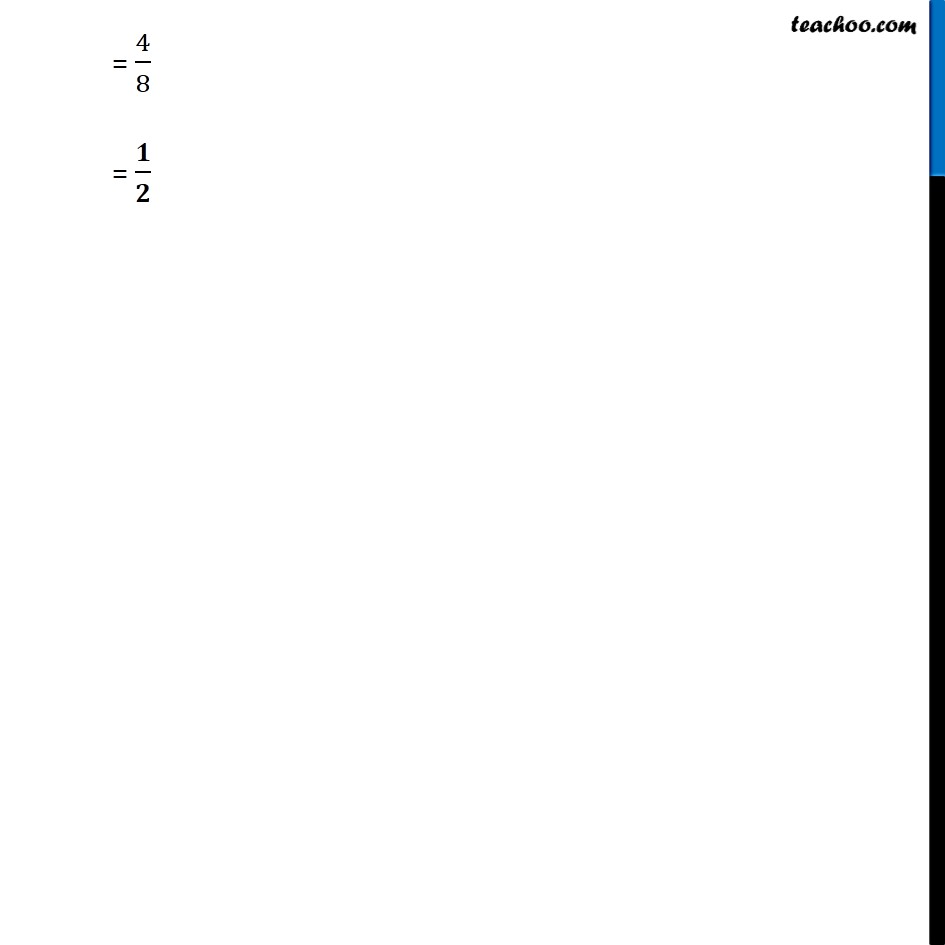1. Chapter 12 Class 8 Exponents and Powers
2. Serial order wise
3. Ex 12.1

Transcript

Ex 12.1, 3 Find the value of. (ii) (2^(−1)× 4^(−1)) ÷〖 2〗^(−2) (2^(−1)× 4^(−1)) ÷〖 2〗^(−2) = (2×4)^(−1) ÷〖 2〗^(−2) = (8)^(−1) ÷〖 2〗^(−2) = 8^(−1)/2^(−2) = 8^(−1)×1/2^(−2) = 1/8^1 ×2^2 = (2 × 2)/8 (𝑈𝑠𝑖𝑛𝑔 𝑎^𝑚×𝑏^𝑚=〖(𝑎×𝑏)〗^𝑚 ) = 4/8 = 𝟏/𝟐